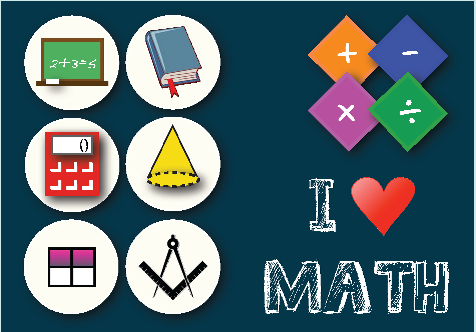# Fourth Grade : Free Math Worksheets

Master place value and number operations. Algebra is introduced in the form of number properties, expressions, writing, and solving basic equations. Focus will be on mastering fractions, decimals, data, graphing, and probability. Get to know about lines, angles, figures and their properties. Learn to measure area and volume of simple figures. The coordinate plane is here too.

Here is the list of all the topics that students learn in this grade. There are some sample worksheets below each section to provide a sense of what to expect. Each section has some free worksheets too. Please subscribe to access the whole content in its best form.We are aligned to common core standards. Click the button below to the see the curriculum.
Common Core

Click on any topic to view, print, or download the worksheets. All of our worksheets are free for non-commercial and personal use.

Featured Content

Points, Lines, Rays, and Angles - I

Identify and differentiate between points, lines and rays. Relate geometry in daily life with these objects. Know the difference between a line and line segment.

Category:  Geometry     Lines, Rays, Angles, and Plane Figures     Points, Lines, Rays and Angles

Equivalent Fractions

Find the equivalent fractions. Create equivalent fractions by shading or coloring the given shapes.

Category:  Fractions     Fractions and Mixed Numbers     Equivalent Fractions